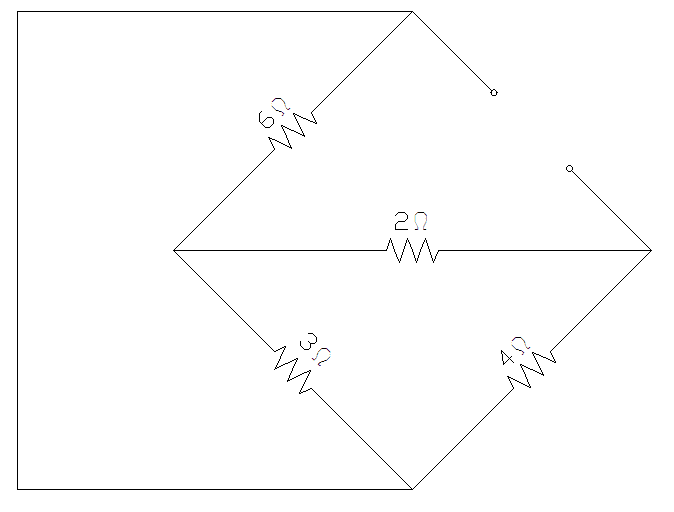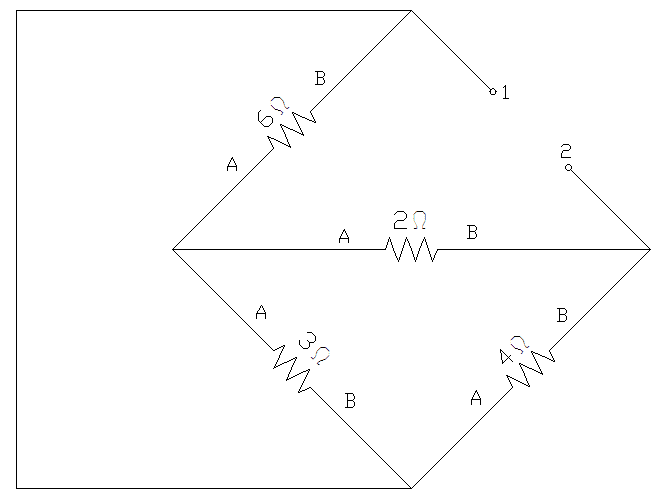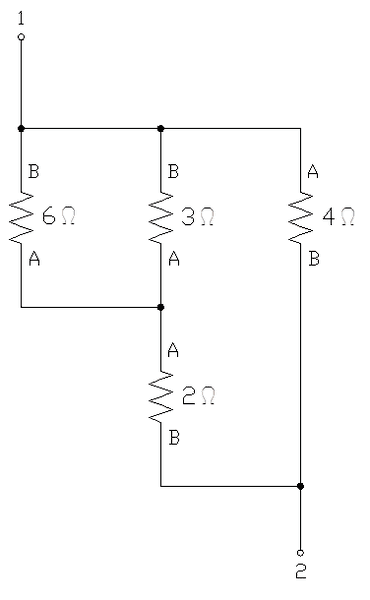# Norton equivalent circuit

• Engineering
I'm having trouble finding the norton equivalent resistance for the attached circuit.

I reasoned that once you short the source, you just get the (3ohm+2ohm) in parallel with the 4ohm resistor.

However the answer i'm getting is not the one given in the book (I get 2.22ohms, when it should be 2ohms). Apparently the 3ohm and the 6ohm resistor are in parallel, I just can't see how. If anyone can guide me through it, you would be doing me a huge favor!

Thanks

#### Attachments

•rnorton.jpg
9 KB · Views: 384

vela
Staff Emeritus
Homework Helper
The 3-ohm+2-ohm combo isn't in parallel with the 4-ohm resistor because the 6-ohm resistor is connected to the node in between the 3-ohm and 2-ohm resistors.

When you short the source, you can drag the top end of the 6-ohm resistor all the way around the loop and down to the bottom, and you'll see that it and the 3-ohm resistor are in parallel.

Fair enough. However I still don't get why the 2ohm and 4ohm resistor are not taken in consideration

vela
Staff Emeritus
Homework Helper
What do you mean?

Well, usually to calculate the thevenin/norton resistance the load is replaced by an open circuit and the source by a short circuit. then i imagine a current entering the circuit from one of the terminals where the load was connected. using this technique, I can't get the correct answer, that is, R_norton = 2ohms

vela
Staff Emeritus
Homework Helper
Oh, I think I understand what you meant. The 6-ohm and 3-ohm resistors combine to give you a 2-ohm resistor, but the fact that the answer to the problem is 2 ohms is just a coincidence. You still have to include the other resistors to get to the final answer.

My guess, is that you're having a hard time visualizing the circuit. Here's a little trick that helps me see simplify the layout:

Starting with the power supply shorted out and the load resistor R removed (open), you have the following:Next, arbitrarily label the ends of each resistor with an "A" on one end and a "B" on the other. Also, label the points between which you are trying to find the total resistance (in this case, the ends where the load resistor used to be attached) as "1" and "2"Now, it's a matter of redrawing the circuit starting with point "1" top and ending with point "2" at the bottom. First, draw point "1" at the top with a line extending out of the bottom. Referring to the last drawing (the original), determine which resistors are connected directly to point 1 ... in this case, the 6-, 3-, and 4-ohm resistors are all connected directly to point 1. So draw 3 branches pointing downward from the line you already drew from the bottom of point 1. Draw one of each of the 3 resistors you located at the bottom of each branch. Now (here's the key), label the top of each of those resistors with the same label as the end of the resistor that connects directly with point 1 (from the original drawing). Label the bottoms of each resistor with the opposite label. Now pick one of the 3 resistors and start from the bottom (open end). Look at the label and find the same end of that resistor in the original drawing ... how many resistors (and/or endpoints) does it connect to? And, to which end (what labels)? Make connections and add branches accordingly. Continue the process until both ends of all resistors have been connected and point 2 has been reached.

Here's how my example came out:It should now be much easier to do your calculations.

zgozvrm, thank you. that was exactly what I was having trouble with. the way you drew it makes it very clear. ((6||3) + 2) || 4 = 2ohms = Rnorton

Thanks a lot for taking the time to do this